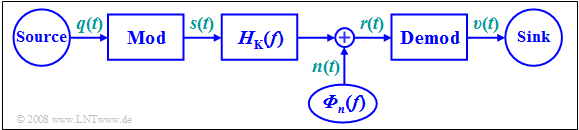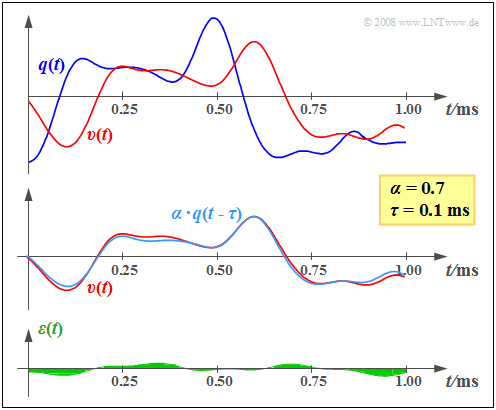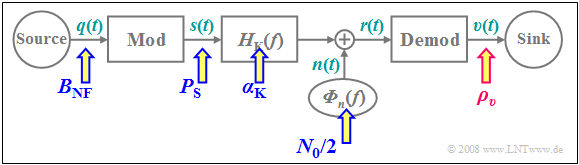# Quality Criteria

## Ideal and distortionless systemBlock diagram describing modulation and demodulation

In all subsequent chapters, the following model will be assumed:

The task of any message transmission system is

• to provide a sink signal  $v(t)$  at a spatially distant sink
• that differs as little as possible from the source signal   $q(t)$ .

$\text{Definition:}$  An  »ideal system«  is achieved when the following conditions hold:

$$v(t) = q(t) + n(t), \hspace{1cm}n(t) \to 0.$$

This takes into account that  $n(t) \equiv 0$  is physically impossible due to  $\text{thermal noise}$.

In practice,  the signals  $q(t)$  and  $v(t)$  will not differ by more than the noise term  $n(t)$  for the following reasons:

• Non-ideal realization of the modulator and the demodulator,
• linear attenuation distortions and phase distortions,  as well as nonlinearities,
• external disturbances and additional stochastic noise processes,
• frequency-independent attenuation and delay.

$\text{Definition:}$  A  »distortionless system«  is achieved,  if from the above list only the last restriction is effective:

$$v(t) = \alpha \cdot q(t- \tau) + n(t), \hspace{1cm}n(t) \to 0.$$

• Due to the attenuation factor  $α$,  the sink signal  $v(t)$ is only  "quieter"  compared to the source signal  $q(t)$.
• Even a delay  $τ$  is often tolerable,  at least for a unidirectional transmission.
• In contrast,  in bidirectional communications – such as a telephone call – a delay of  $300$  milliseconds is already perceived as a significant disturbance.

## Signal–to–noise (power) ratio

In the general case,  the sink signal  $v(t)$  will still differ from  $α · q(t - τ)$,  and the error signal is characterized by:

$$\varepsilon (t) = v(t) - \alpha \cdot q(t- \tau) = \varepsilon_{\rm V} (t) + \varepsilon_{\rm St} (t).$$

This error signal is composed of two components:

• linear and nonlinear distortions  (German:  "Verzerrungen"   ⇒   subscript "V")  $ε_{\rm V}(t)$,  which are caused by the frequency responses of the modulator,  the channel,  and the demodulator and thus exhibit deterministic  (time-invariant)  behavior;
• a stochastic component $ε_{\rm St}(t)$,  which originates from the high-frequency noise   $n(t)$  at the demodulator input.  However,  unlike   $n(t)$,  $ε_{\rm St}(t)$  is usually due to a low-frequency noise disturbance in a demodulator with a low-pass characteristic curve.

$\text{Definition:}$  As a measure of the quality of the communication system,  the  »signal-to-noise (power) ratio«  $\rm (SNR)$  $ρ_v$  at the sink is defined as the quotient of the signal power (variance) of the useful component  $v(t) - ε(t)$  and the disturbing component  $ε(t)$,  respectively:

$$\rho_{v} = \frac{ P_{v -\varepsilon} }{P_{\varepsilon} } \hspace{0.05cm},\hspace{0.7cm}\text{with}\hspace{0.7cm} P_{v -\varepsilon} = \overline{[v(t)-\varepsilon(t)]^2} = \lim_{T_{\rm M} \rightarrow \infty}\hspace{0.1cm}\frac{1}{T_{\rm M} } \cdot \int_{0}^{ T_{\rm M} } {\big[v(t)-\varepsilon(t)\big]^2 }\hspace{0.1cm}{\rm d}t,\hspace{0.5cm} P_{\varepsilon} = \overline{\varepsilon^2(t)} = \lim_{T_{\rm M} \rightarrow \infty}\hspace{0.1cm}\frac{1}{T_{\rm M} } \cdot \int_{0}^{ T_{\rm M} } {\varepsilon^2(t) }\hspace{0.1cm}{\rm d}t\hspace{0.05cm}.$$

For the power of the useful part,  we obtain regardless of the delay time  $τ$:

$$P_{v -\varepsilon} = \overline{\big[v(t)-\varepsilon(t)\big]^2} = \overline{\alpha^2 \cdot q^2(t - \tau)}= \alpha^2 \cdot P_{q}.$$

Here,  $P_q$  denotes the power of the source signal  $q(t)$:

$$P_{q} = \lim_{T_{\rm M} \rightarrow \infty}\hspace{0.1cm}\frac{1}{T_{\rm M}} \cdot \int_{0}^{ T_{\rm M}} {q^2(t) }\hspace{0.1cm}{\rm d}t .$$

This gives:

$$\rho_{v} = \frac{\alpha^2 \cdot P_{q} }{P_{\varepsilon} } \hspace{0.3cm}\Rightarrow \hspace{0.3cm} 10 \cdot {\rm lg}\hspace{0.15cm}\rho_{v} = 10 \cdot {\rm lg} \hspace{0.15cm} \frac{\alpha^2 \cdot P_{q} }{P_{\varepsilon} } \hspace{0.05cm}.$$
• In the following,  we will refer to  $ρ_v$  as the  »sink signal–to–noise ratio«   or short:  »sink SNR«.
• One often uses the logarithmic form   ⇒   $10 · \lg \ ρ_v$  which is expressed in  $\rm dB$  when using the logarithm of base ten   $(\lg)$ .Illustrating the remaining error signal  $ε(t) = v(t) - α · q(t - τ)$

$\text{Example 1:}$  On the right, you can see an exemplary section of the  (blue)  source signal  $q(t)$  and the  (red)  sink signal  $v(t)$, which are noticeably different.

However, the middle graph makes it clear that the main difference between  $q(t)$  and  $v(t)$  is due to the attenuation factor  $α = 0.7$  and the transmission delay  $τ = 0.1\text{ ms}$.

The bottom sketch shows the remaining error signal  $ε(t) = v(t) - α · q(t - τ)$  after correcting for attenuation and delay.  We refer to the mean square ⇒ "variance" of this signal as the noise power  $P_ε$.

To calculate the sink SNR  $ρ_v$ ,  $P_ε$  must be related to the useful signal power  $α^2 · P_q$.  This is obtained from the variance of the signal  $α · q(t - τ)$,  plotted in light blue in the middle graph.

From the assumed properties  $\alpha = 0.7$   ⇒   $\alpha^2 \approx 0.5$  as well as  $P_{q} = 8\,{\rm V^2}$  and  ${P_{\varepsilon} } = 0.04\,{\rm V^2}$ , we obtain the sink SNR

$$ρ_v ≈ 100 \hspace{0.3cm}\Rightarrow \hspace{0.3cm}10 · \lg ρ_v ≈ 20\ \rm dB.$$
• The error signal  $ε(t)$  – and thus also the sink SNR  $ρ_v$  – takes into account all imperfections of the transmission system under consideration (e.g. distortions, external interferences, noise, etc.).
• In the following,  we will consider each of these different effects separately for the sake of explanation.

## Investigations with regard to signal distortions

All modulation methods described in the following chapters lead to distortions under non-ideal conditions, i.e. to a sink signal

$$v(t) ≠ α · q(t - τ),$$

which differs from  $q(t)$  by more than just attenuation and delay.  For the study of these signal distortions, we always assume the following model and premises:

• The additive noise signal  $n(t)$  at the channel output  (demodulator input)  is negligible and ignored.
• All components of modulator and demodulator are treated as linear.
• Similarly,  the channel is assumed to be linear,  and is thus completely characterized by its frequency response  $H_{\rm K}(f)$ .

Depending on the type and realization of modulator and demodulator, the following signal distortions occur:

$\text{Linear distortions}$, as described in the  "chapter of the same name"  in the book "Linear and Time-Invariant Systems":

• Linear distortions can generally be compensated by an equalizer,  but this will always result in higher  $P_\epsilon$  and thus in a lower sink SNR in the presence of a stochastic disturbance  $n(t)$.
• These linear distortions can be further divided into  "attenuation distortions"  and  "phase distortions".

$\text{Nonlinear distortions}$, as described in the  "chapter of the same name"  in the book  "Linear and Time-Invariant Systems":

• Nonlinear distortions are irreversible and thus a more severe problem than linear distortions.
• A suitable quantitative measure of such distortions is the distortion factor  $K$,  for example, which is related to the sink SNR in the following way:   $\rho_{v} = {1}/{K^2} \hspace{0.05cm}.$
• However,  specifying a distortion factor assumes a harmonic oscillation as the source signal.

We refer you to three of our  (German language)  basic learning videos:

$\text{Two further points:}$

1.   The distortions with respect to  $q(t)$  and  $v(t)$  are nonlinear in nature whenever the channel contains nonlinear components and, as such,
nonlinear distortions are already present with respect to the signals  $s(t)$  and  $r(t)$.
2.   Similarly,  nonlinearities in the modulator or demodulator always lead to nonlinear distortions.

## Some remarks on the AWGN channel model

To investigate the noise behavior of each individual modulation and demodulation method, the starting point is usually the so-called  $\rm AWGN$  channel, where the abbreviation stands for  "$\rm A$dditive $\rm W$hite $\rm G$aussian $\rm N$oise".  The name already sufficiently describes the properties of this channel model.

We would also like to refer you to the  (German language)  three-part learning video  "Der AWGN-Kanal"   ⇒   "The AWGN channel".

• The additive noise signal includes all frequency components equally   ⇒   $n(t)$  has a constant power-spectral density   $\rm (PSD)$ and a Dirac-shaped auto-correlation function $\rm (ACF)$:
$${\it \Phi}_n(f) = \frac{N_0}{2}\hspace{0.15cm} \bullet\!\!-\!\!\!-\!\!\!-\!\!\circ\, \hspace{0.15cm} \varphi_n(\tau) = \frac{N_0}{2} \cdot \delta (\tau)\hspace{0.05cm}.$$
In each case,  the factor  $1/2$  in these equations accounts for the two-sided spectral representation.
• For example,  in the case of thermal noise,  for the physical noise power density  (from a one-sided view)  with a noise figure  $F ≥ 1$  and an absolute temperature  $θ$:
$${N_0}= F \cdot k_{\rm B} \cdot \theta , \hspace{0.5cm}\text{Boltzmann constant:}\hspace{0.3cm}k_{\rm B} = 1.38 \cdot 10^{-23}{ {\rm Ws} }/{ {\rm K} }\hspace{0.05cm}.$$
• "True white noise"  would result in infinitely large power.  Therefore,  a bandwidth limit of  $B$  must always be taken into account,  and the following applies to the effective noise power:
$$N = \sigma_n^2 = {N_0} \cdot B \hspace{0.05cm}.$$
• The noise signal  $n(t)$  has a Gaussian probability density function $\rm (PDF)$   ⇒ a normal amplitude distribution with standard deviation  $σ_n$:
$$f_n(n) = \frac{1}{\sqrt{2\pi}\cdot\sigma_n}\cdot {\rm e}^{-{\it n^{\rm 2}}/{(2\sigma_{\it n}^2)}}.$$
• For the AWGN channel,  one should actually set  $H_{\rm K}(f) = 1$.  However,  we modify this model for our purposes by allowing frequency-independent attenuation
(note:  a frequency-independent attenuation factor does not lead to further distortions):
$$H_{\rm K}(f) = \alpha_{\rm K}= {\rm const.}$$

## Investigations at the AWGN channel

In all investigations regarding noise behavior, we start from the block diagram sketched below. We will always calculate the sink SNR  $ρ_v$  as a function of all system parameters and arrive at the following results:Block diagram for investigating noise behavior
• The more transmit power  (German:  "Sendeleistung"   ⇒   subscript "S")   $P_{\rm S}$  we apply,  the greater is the sink SNR  $ρ_v$.  For some methods,  this relationship can even be linear.
• $ρ_v$  decreases monotonically with increasing noise power density  $N_0$ .  An increase in  $N_0$  can usually be compensated by a larger transmit power  $P_{\rm S}$.
• The smaller the channel's  $α_{\rm K}$ parameter,  the smaller  $ρ_v$ becomes.  There is often a quadratic relationship, since the received power  (German:  "Empfangsleistung"   ⇒ subscript "E")   is  $P_{\rm E} = {α_{\rm K}}^2 · P_{\rm S}$.
• A wider bandwidth of the source signal $($larger  $B_{\rm NF})$  requires an increased high-frequency bandwidth $B_{\rm HF}$,  too   ⇒   this leads to smaller sink SNR  $ρ_v$   ⇒   negative effect on the transmission system's quality.

$\text{Conclusion:}$  Considering these four assumptions, we conclude that it makes sense to express the sink SNR in normalized form as

$$\rho_{v } = \rho_{v }(\xi) \hspace{0.5cm} {\rm with} \hspace{0.5cm}\xi = \frac{ {\alpha_{\rm K} }^2 \cdot P_{\rm S} }{N_0 \cdot B_{\rm NF} }.$$

In the following,  we refer to   $ξ$  as the  »performance parameter«.

The input variables summarized in  $ξ$  are marked with blue arrows in the above block diagram, while the quality criterion  $ρ_v$  is highlighted by the red arrow.

• The larger  $ξ$  is,  the larger is   $\rho_{v }$ in general.
• But the relationship is not always linear,  as the following example shows.

$\text{Example 2:}$  The left graph shows the sink SNR  $ρ_v$  of three different systems,  each as a function of the normalized performance parameter

$$\xi = { {\alpha_{\rm K} }^2 \cdot P_{\rm S} }/({N_0 \cdot B_{\rm NF} }).$$
• For  $\text{System A}$,  $ρ_ν = ξ$  holds.  The system parameters
$$P_{\rm S}= 10 \;{\rm kW}\hspace{0.05cm}, \hspace{0.2cm} \alpha_{\rm K} = 10^{-4}\hspace{0.05cm},$$
$${N_0} = 10^{-12}\hspace{0.05cm}{ {\rm W} }/{ {\rm Hz} }\hspace{0.05cm}, \hspace{0.2cm} B_{\rm NF}= 10\; {\rm kHz}$$
give  $ξ = ρ_v = 10000$  (see the circular mark on the graph).
• Exactly the same sink SNR would result from the parameters
$$P_{\rm S}= 5 \;{\rm kW}\hspace{0.05cm}, \hspace{0.2cm} \alpha_{\rm K} = 10^{-6}\hspace{0.05cm},$$
$${N_0} = 10^{-16}\hspace{0.05cm}{ {\rm W} }/{ {\rm Hz} }\hspace{0.05cm}, \hspace{0.2cm} B_{\rm NF}= 5\; {\rm kHz}\hspace{0.05cm}.$$
• In  $\text{System B}$,  there is also a linear relationship of  $ρ_v = ξ/3$.  The line also passes through the origin.  However, the slope is only  $1/3$.
• It should be noted that the noise behavior corresponding to  $\text{System A}$  is observed for   $\text{double-sideband suppressed-carrier amplitude modulation}$  $($modulation depth  $m → ∞)$,  while  $\text{System B}$  describes   $\text{double-sideband amplitude modulation with carrier}$  $(m ≈ 0.5)$.
• $\text{System C}$  shows a completely different noise behavior.  For small  $ξ$–values,  this system is superior to  $\text{System A}$,  though the quality of both systems is the same at  $ξ = 10000$.

Increasing the performance parameter  $ξ$  does not significantly improve  $\text{System C}$,  unlike in $\text{System A}$.  Such behavior can be observed,  for example,  in digital systems where the sink SNR is limited by the quantization noise.  Along the horizontal section of the curve,  a higher transmit power will not result in a better sink SNR – and thus a smaller bit error probability.

Usually,  the quantities  $ρ_v$  and  $ξ$  are represented in logarithmic form,  as shown in the graph on the right:

• The double logarithmic representation still results in the angle bisector for  $\text{System A}$ .
• The lower slope  $($factor $3)$  of  $\text{System B}$  now results in a downward shift of  $10 · \lg 3 ≈ 5\text{ dB}$.
• The intersection of  $\text{A}$  and  $\text{C}$  shifts from  $ξ = ρ_v = 10000$  to  $10 · \lg ξ = 10 · \lg ρ_v = 40\text{ dB}$ due to the double-logarithmic representation.